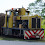## Monday, April 04, 2016

### Are perfect numbers bigger than 6 initial sums of odd cubes?

I pose two questions today (Monday April 4).

I will post the answers tomorrow (Tuesday April 5).

If I need to clarify something I will do it in the main post So, to reiterate- feel free to leave spoilers but if you want to avoid reading them, don't read the comments.

Note:

The first four perfect numbers are 6, 28, 496, 8128

28 = 13 + 33

496 = 13 + 33 + 53 + 73

Is 8128 the sum of the first six odd cubes? No, and that is not one of my questions.

Questions:

1) Is there a k such that 8128  is the sum of the first k odd cubes?

2) Is there something interesting going on here?

1.Let sum(k) is the sum of the first k odd cubes. sum(2) = 28, sum(4) = 496, sum(8) = 8128. This gets more interesting when we find that sum(64) = 33550336, sum(256) = 8589869056 and sum(512) = 137438691328.

2.One would think that two raised to an odd prime happens to be equal to a square doubled...

3.Jordan Schneider12:20 PM, April 04, 2016

1) k = 8 gives you 8128.
2) There's something going on with powers of two. k = 64 gives you 33550336, the next perfect number. k = 256 gives you 8589869056, the one after that. 2, 4, 8, 64, 256 are all powers of two, but there's no clear closed form. I can't find anything particularly interesting about the powers of two in between. I may come back to this later.

4.Even perfect numbers k greater than 6 are of the form 2^(p-1)*(2^p - 1). Then, according to Wikipedia, k is the sum of the first 2^((p-1)/2) odd cubes.

5.Here's what's going on:

First, note that the sum of the sum of the first N odd cubes is exactly N^2(2N^2-1). You can prove this from scratch or from known identities, like the fact that the sum of the first n cubes is (n^2+n)^2/4. (The fact that this is a square makes people rather happy: https://en.wikipedia.org/wiki/Squared_triangular_number .) But, the easiest way is just to note that the result must be a degree four polynomial and then just interpolate.

The interesting behavior observed in the OP then follows from the Euclid-Euler Theorem, which states that M is an even perfect number iff M = 2^{p-1} * (2^p -1), where 2^p - 1 is prime. (See here for a proof: https://en.wikipedia.org/wiki/Euclid%E2%80%93Euler_theorem. I like the fact that Euclid and Euler apparently managed to collaborate across millenia :).) We may assume without loss of generality that p is a prime as well, since otherwise 2^p - 1 has non-trivial factors. Note that for p > 2, we can also write M = N^2(2N^2 - 1) by simply substituting N = 2^{(p-1)/2}. (Since p is a prime greater than 2, p is odd, so (p-1)/2 is an integer.)

So, all even perfect numbers other than 6 can be written as the sum of the first N odd perfect cubes. And, the sum of the first N odd primes is an even perfect number if and only if N is a power of two AND 2N^2 - 1 is prime.

6.This is known fact...look it up in Wikipedia!

7.What is interesting is whether you can use the fact that perfect numbers are the sum of the cubes of an initial segment of odd numbers to prove that there are infinitely many even perfects. In that case, it follows also that there are infinitely many Marsenne primes